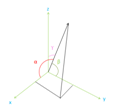## Three Dimensional Vector Angle Calculation

Calculate the angle of three dimensional vectors (3D Vectors) with entered vector coordinates. The 3D vectors are using the x-y-z axes.
α is the angle between u and the x-axis.
β is the angle between u and the y-axis.
γ is the angle between u and the z-axis.Vector V1: ,, Vector V2: ,, Angle: Degrees